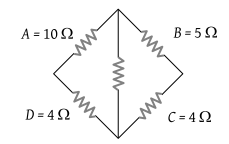# NEET Questions Solved

In a typical Wheatstone network, the resistances in cyclic order are A = 10 Ω, B = 5 Ω, C = 4 Ω and D = 4 Ω for the bridge to be balanced(1) 10 Ω should be connected in parallel with A

(2) 10 Ω should be connected in series with A

(3) 5 Ω should be connected in series with B

(4) 5 Ω should be connected in parallel with B

(1) For a balance Wheatstone bridge.

$\frac{A\text{'}}{B}=\frac{D}{C}⇒\frac{A\text{'}}{5}=\frac{4}{4}⇒A\text{'}=5\Omega$

$A\text{'}\left(5\Omega \right)$ is obtained by connecting a $10\text{\hspace{0.17em}}\Omega$ resistance in parallel with A.

Difficulty Level:

• 47%
• 18%
• 28%
• 9%